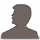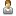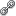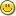# The Equation

Komentáře k článku: The Equation

1=2

I know something similar
just for fun:-)ENJOY

1=2: A Proof using Beginning Algebra

1. Let a=b.
2. Then aa=ab,
3. aa + aa = aa + ab,
4. 2aa = aa + ab,
5. 2aa – 2ab = aa + ab – 2ab,
6. and 2aa – 2ab = aa – ab
7. This can be written as
2(aa – ab) = 1(aa – ab)
8. and cancelling the
(aa – ab)
from both sides
gives us 1=2Neregistrovaný uživatelPříspěvek od Mato37 vložený před 13 lety

1=2

I know something similar
just for fun:-)ENJOY

1=2: A Proof using Beginning Algebra

1. Let a=b.
2. Then aa=ab,
3. aa + aa = aa + ab,
4. 2aa = aa + ab,
5. 2aa – 2ab = aa + ab – 2ab,
6. and 2aa – 2ab = aa – ab
7. This can be written as
2(aa – ab) = 1(aa – ab)
8. and cancelling the
(aa – ab)
from both sides
gives us 1=2

Re: 1=2

The only thin is that if a=b, then (aa-ab)=0.
So in the last step, you actually dividing whole equation by zero, something that is not permitted and causes this whole riddicolous equality.Příspěvek od Neregistrovaný uživatel vložený před 13 lety

Re: 1=2

The only thin is that if a=b, then (aa-ab)=0.
So in the last step, you actually dividing whole equation by zero, something that is not permitted and causes this whole riddicolous equality.

Re: Re: 1=2

I wouldn't call this equation ridiculous.
Well, dividing by zero may not be permitted, it may even be impossible but despite this I think Mato37's compu­tation looks quite charming and, at first sight, even mysterious…I like it.

And I like „The Equation“ as well! Thanks for it.

42

Trochu mi to připomíná „The Hitchhiker's Guide to the Galaxy“ od Douglase Adamse, kde je zase zjištěno, že „Answer to the Ultimate Question of Life, the Universe, and Everything“ je 42Pro zájemce o spojitost mezi matematikou(lo­gikou) a filosofií doporučuji Gödelovy věty o neúplnosti, naprosto fascinující!

Re: Re: Re: 1=2It looks charming for people who don't understand mathematics. You can do a lot of „charming“ things similar way.

Příspěvky mohou přidávat pouze přihlášení uživatelé. Pokud máte účet můžete se přihlásit.

Příspěvky v diskusi jsou majetkem jejich autorů. Provozovatel webových stránek Help For English za ně nenese zodpovědnost.